﻿ A Two-Parameter Poisson-Akash Distribution with Properties and ApplicationsInternational Journal of Probability and Statistics

p-ISSN: 2168-4871    e-ISSN: 2168-4863

2018;  7(4): 114-123

doi:10.5923/j.ijps.20180704.03A Two-Parameter Poisson-Akash Distribution with Properties and Applications

Rama Shanker1, Kamlesh Kumar Shukla1, Tekie Asehun Leonida2

1Department of Statistics, College of Science, Eritrea Institute of Technology, Asmara, Eritrea

2Department of Applied Mathematics, University of Twente, The Netherlands

Correspondence to: Rama Shanker, Department of Statistics, College of Science, Eritrea Institute of Technology, Asmara, Eritrea.
 Email: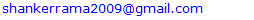Abstract

This paper proposes a two-parameter Poisson- Akash distribution which includes Poisson-Akash distribution as a special case. Its moments and moments based measures have been derived and studied. Statistical properties including hazard rate function, unimodality and generating functions have been discussed. Method of moments and the method of maximum likelihood have been discussed for estimating the parameters of the distribution. Finally, applications of the proposed distribution have been explained through two count datasets from biological sciences and compared with other discrete distributions.

Keywords: Two-parameter Akash distribution, Poisson- Akash distribution, Compounding, Moments, Skewness, Kurtosis, Maximum likelihood estimation, Applications

Cite this paper: Rama Shanker, Kamlesh Kumar Shukla, Tekie Asehun Leonida, A Two-Parameter Poisson-Akash Distribution with Properties and Applications, International Journal of Probability and Statistics , Vol. 7 No. 4, 2018, pp. 114-123. doi: 10.5923/j.ijps.20180704.03.

1. Introduction

The modeling and statistical analysis of count data are crucial in almost every fields of knowledge including biological science, insurance, medical science, and finance, some amongst others. Count data are generated by various phenomena such as the number of insurance claimants in insurance industry, number of yeast cells in biological science, number of chromosomes in genetics, etc. It has been observed that, in general, count data follows under-dispersion (variance < mean), equi-dispersion (variance = mean) or over-dispersion (variance > mean). The over-dispersion of count data have been addressed using mixed Poisson distributions by different researchers including Raghavachari et al (1997), Karlis and Xekalaki (2005), Panjeer (2006), are some among others. Mixed Poisson distributions arise when the parameter of the Poisson distribution is a random variable having some specified distributions. The distribution of the parameter of the Poisson distribution is known as mixing distribution. It has been observed that the general characteristics of the mixed Poisson distribution follow some characteristics of its mixing distributions. In distribution theory, various mixed Poisson distributions have been derived by selecting a proper mixing distribution.
The classical negative binomial distribution (NBD) derived by Greenwood and Yule (1920) is the mixed Poisson distribution where the mean of the Poisson random variable is distributed as a gamma random variable. The NBD has been used to model over-dispersed count data. However, the NBD may not be appropriate for some over-dispersed count data due to its theoretical or applied point of view. Other mixed Poisson distributions arise from the choice of alternative mixing distributions. For example, the Poisson-Lindley distribution, introduced by Sankaran (1970), is a Poisson mixture of Lindley (1958) distribution. The Poisson-Akash distribution, introduced by Shanker (2017), is a Poisson mixture of Akash distribution suggested by Shanker (2015). It has been observed by Karlis and Xekalaki (2005) that there are naturally situations where a good fit is not obtainable with a particular mixed Poisson distribution in case of over-dispersed count data. This shows that there is a need for new mixed Poisson distribution which gives a better fit as compared with the existing mixed Poisson distributions.
Shanker (2017) proposed the discrete Poisson- Akash distribution (PAD) to model count data defined by its probability mass function (pmf)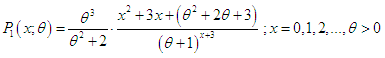(1.1)
Moments and moments based measures, statistical properties; estimation of parameter using both the method of moments and the method of maximum likelihood and applications of PAD has been discussed by Shanker (2017). The distribution arises from the Poisson distribution when its parameter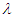follows Akash distribution introduced by Shanker (2015) and defined by its probability density function (pdf)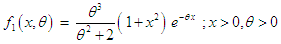(1.2)
The pdf (1.2) is a convex combination of exponential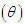and gamma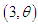distributions. Shanker (2015) discussed statistical properties including moments based coefficients, hazard rate function, mean residual life function, mean deviations, stochastic ordering, Renyi entropy measure, order statistics, Bonferroni and Lorenz curves, stress- strength reliability, along with estimation of parameter and applications to model lifetime data from biomedical science and engineering.
The first four moments about origin and the variance of PAD (1.1) obtained by Shanker (2017) are given by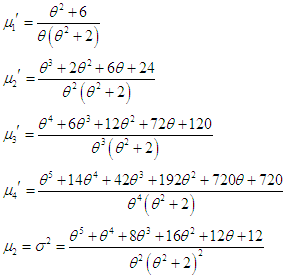Shanker and Shukla (2017) proposed a two-parameter Akash distribution (TPAD) having parameters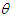and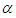and defined by its pdf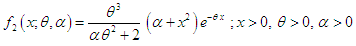(1.3)
Its structural properties including moments, hazard rate function, mean residual life function, mean deviations, stochastic ordering, Renyi entropy measure, order statistics, Bonferroni and Lorenz curves, stress- strength reliability , estimation of parameters and applications for modeling survival time data has been discussed in Shanker and Shukla (2017). It can be easily shown that at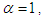TPAD (1.3) reduces to Akash distribution (1.2).
The main purpose of this paper is to propose a two-parameter Poisson- Akash distribution, a Poisson mixture of two-parameter Akash distribution suggested by Shanker and Shukla (2017). Its moments based measures including coefficients of variation, skewness, kurtosis and index of dispersion have been derived and their behaviors have been discussed graphically. Its statistical properties including hazard rate function, unimodality and generating functions have been studied. The estimation of parameters has been discussed using method of moments and the method maximum likelihood. Applications and goodness of fit of the distribution has also been discussed through two examples of observed real count datasets from biological sciences and the fit has been compared with other discrete distributions.

2. A Two-Parameter Poisson-Akash Distribution

Assuming that the parameter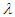of the Poisson distribution follows TPAD (1.3), the Poisson mixture of TPAD can be obtained as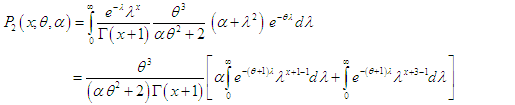(2.1)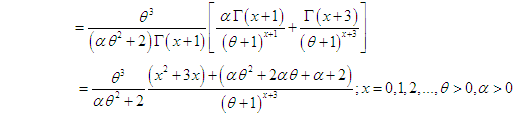(2.2)
We would call this pmf a two-parameter Poisson - Akash distribution (TPPAD). It can be easily verified that PAD (1.1) is a particular case of TPPAD for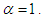The nature and behavior of TPPAD for varying values of the parameters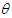and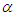have been explained graphically in figure 1.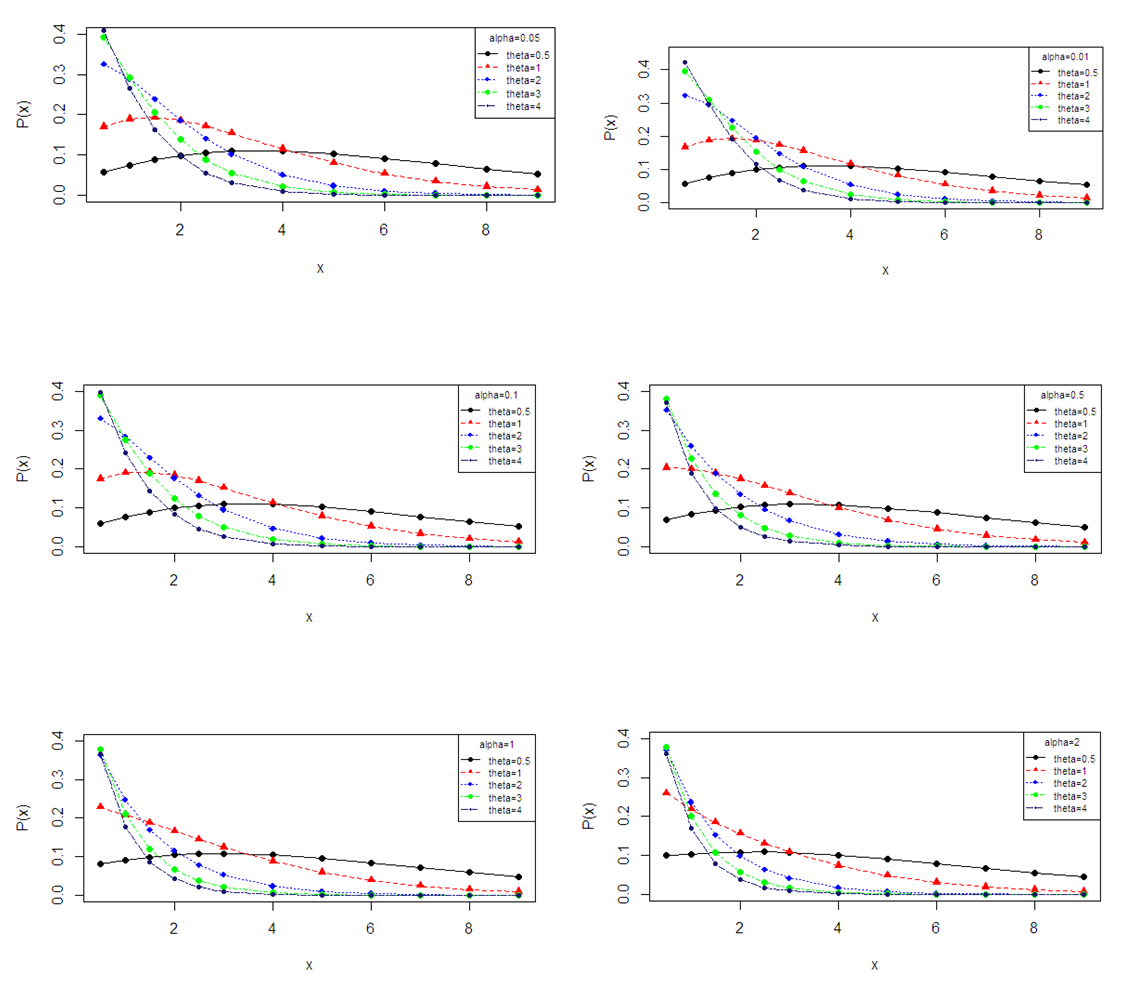Figure 1. Probability mass function plot of TPPAD for varying values of parameters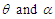3. Statistical Constants

In this section factorial moments, raw moments, central moments and moments based statistical measures including coefficient of variation, skewness, kurtosis and index of dispersion of TPPLD has been obtained.

3.1. Factorial Moments

Using (2.1), the th factorial moment about origin of the TPPAD (2.2) can be obtained as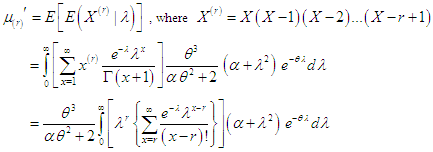Assuming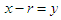, we get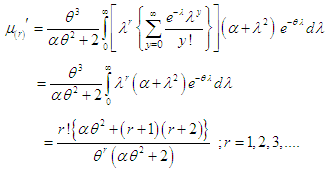(3.1.1)
Taking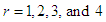in (3.1.1), the first four factorial moments about origin of TPPAD (2.2) can be obtained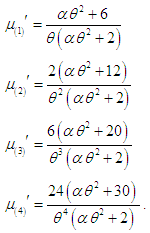3.2. Raw Moments (Moments about Origin)

Using the relationship between factorial moments about origin and the raw moments, the first four raw moments of TPPAD (2.2) can be obtained as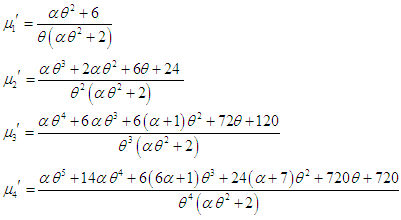3.3. Central Moments (Moments about Mean)

Using the relationship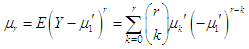between central moments and the raw moments, the central moments of the TPPAD (2.2) can be obtained as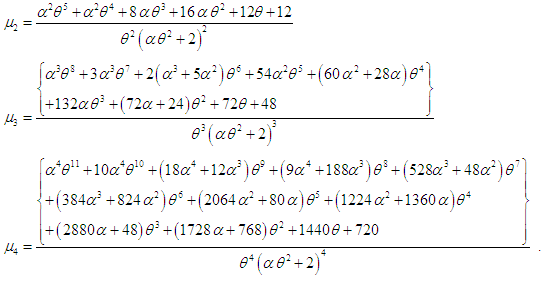3.4. Coefficients of Variation, Skewness, Kurtosis and Index of Dispersion

The coefficient of variation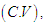coefficient of Skewness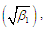coefficient of Kurtosis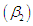and index of dispersion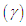of the TPPAD (2.2) are thus obtained as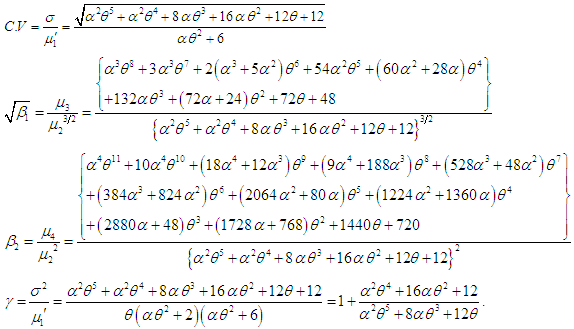Now from the index of dispersion it is obvious that if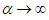and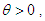then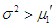(over dispersion); if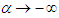and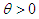, then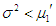(under dispersion) and if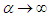and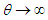, then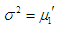(equi dispersion). Nature and behavior of coefficient of variation, coefficient of skewness, coefficient of kurtosis and index of dispersion of TPPAD for varying values of parameters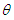and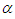have been shown graphically in figure 2.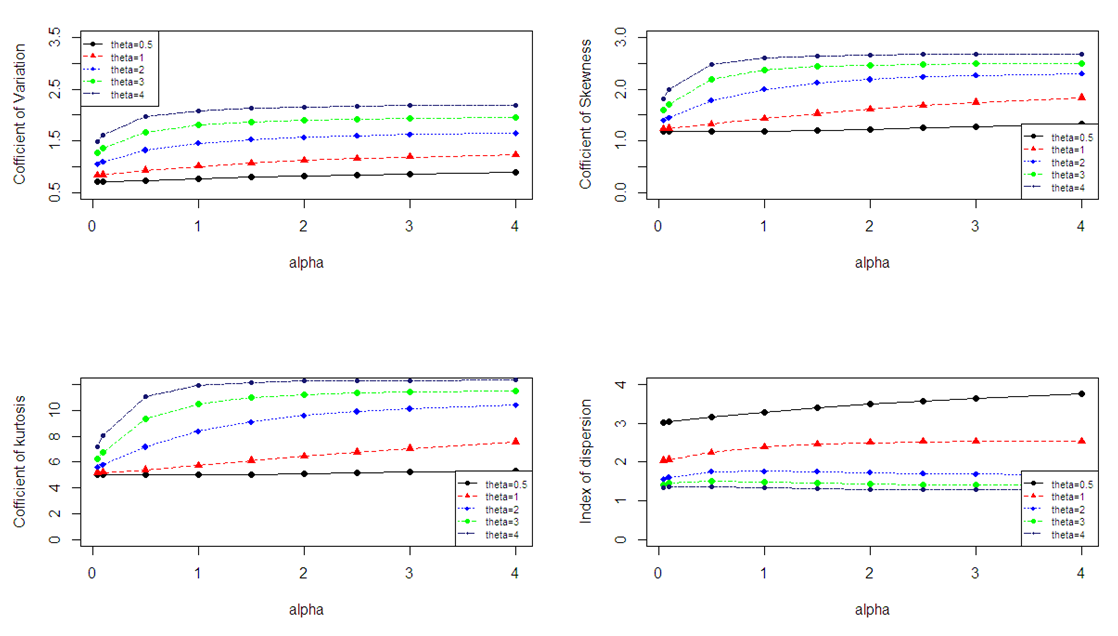Figure 2. Nature and behavior of coefficient of variation, coefficient of skewness, coefficient of kurtosis and index of dispersion of TPPAD for varying values of parameters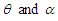4. Statistical Properties

In this section the unimodality, increasing hazard rate, probability generating function and the moment generating function of TPPLD has been discussed.

4.1. Increasing Hazard Rate and Unimodality

We have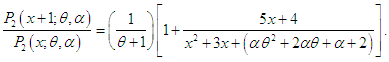It can be easily verified that this is a decreasing function in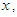and hence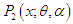is log-concave. Now using the results of relationship between log-concavity, unimodality and increasing hazard rate (IHR) of discrete distributions given in Grandell (1997), it can concluded that TPPAD (2.2) has an increasing hazard rate and is unimodal.

4.2. Generating Functions

The probability generating function of TPPAD can be obtained as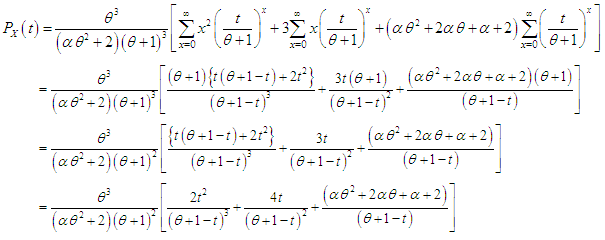The moment generating function of TPPAD is thus given by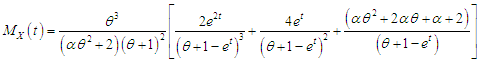5. Estimation of Parameters

In this section the estimation of parameters of TPPLD using the method of moments and the method of maximum likelihood has been discussed.

5.1. Method of Moments Estimation of Parameters

Since TPPAD has two parameters to be estimated, taking the first two moments about origin, we have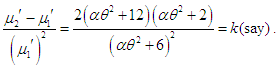Assuming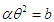, we get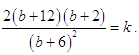This gives a quadratic equation in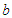as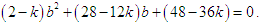Replacing the first population moment about origin and the second population moment about origin with their respective sample moments, an estimate of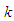can be obtained and substituting the value of in the above equation, an estimate of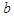can be obtained. Again, replacing the population mean with the corresponding sample mean and taking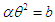in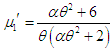, we get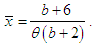This gives the method of moments estimate (MOME)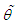of parameter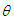as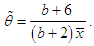Thus the MOME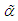of parameter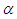is given by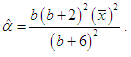5.2. Maximum Likelihood Estimation of Parameters

Suppose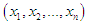be a random sample of size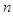from the TPPAD (2.2) and let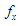be the observed frequency in the sample corresponding to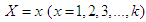such that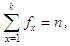where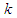is the largest observed value having non-zero frequency. The log- likelihood function of TPPAD (2.2) can be given by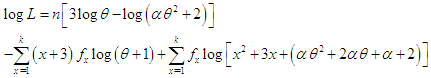The maximum likelihood estimates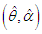of parameters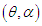of TPPAD (2.2) is the solutions of the following log- likelihood equations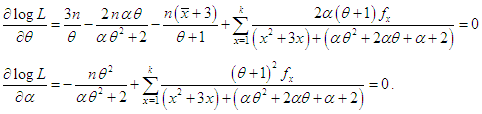whereis the sample mean. These two log likelihood equations do not seem to be solved directly because they are not in closed forms. These two log-likelihood equations can be solved iteratively using R-software till sufficiently close estimates of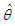and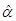are obtained.

6. Applications

The TPPAD has been fitted to two count datasets from biological sciences to test its goodness of fit over Poisson distribution (PD), Poisson-Lindley distribution (PLD) and Poisson-Akash distribution (PAD) using maximum likelihood estimates (MLE’s) of parameters. The first dataset is the number of Student’s historic data on Haemocytometer counts of yeast cells available in Gosset (1908) and the second data set is the number of European corn- borer of Mc. Guire et al (1957). The fitted plots of distributions for datasets in tables 1 and 2 are presented in figure 3. Since the expected frequencies given by TPPAD are more closure to the original frequencies than the expected frequencies given by PD, PLD, and PAD, it is clear from the goodness of fit of TPPAD and from the fitted plots of distributions that TPPAD gives much closer fit than PD, PLD, and PAD and hence it can be considered as an important distribution in ecology.
 Table 1. Observed and expected number of Haemocytometer yeast cell counts per square observed by Gosset (1908)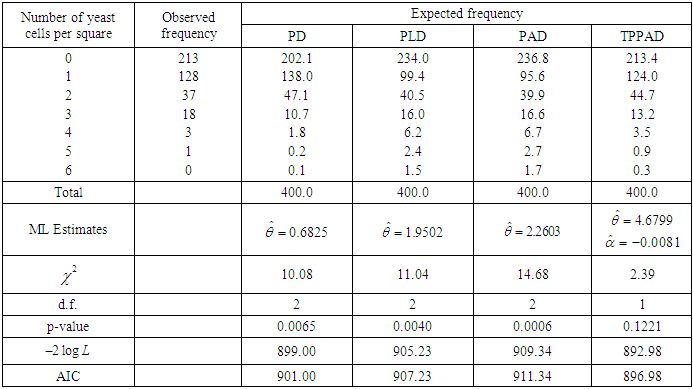Table 2. Observed and expected number of European corn- borer of Mc. Guire et al (1957)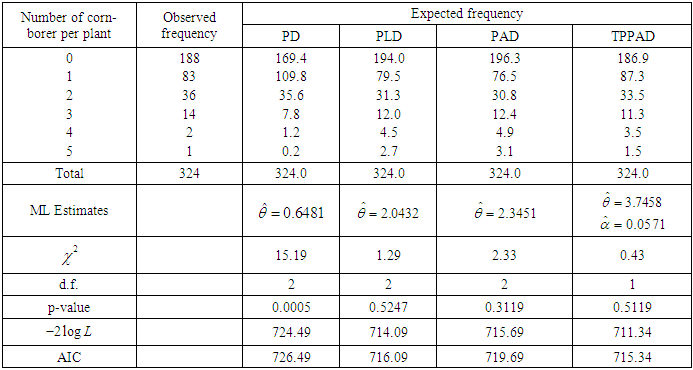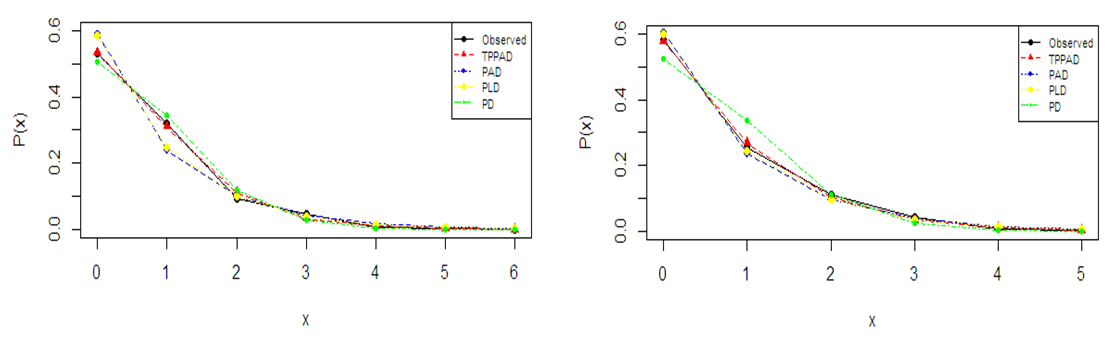Figure 3. Fitted plots of distributions for datasets 1 and 2

7. Conclusions

A two-parameter Poisson-Akash distribution (TPPAD) which includes one parameter Poisson-Akash distribution has been proposed. Its moments and moments based statistical constants have been derived and studied. Some statistical properties have been discussed. Method of moments and the method of maximum likelihood have been discussed for estimating parameters of the distribution. Finally, applications of the proposed distribution have been explained with two count datasets from biological sciences and fit has been found quite satisfactory over other discrete distributions including PD, PLD and PAD.

ACKNOWLEDGEMENTS

Authors are grateful to the editor in chief of the journal and the anonymous reviewer for their fruitful comments.

References

  Gosset, W.S. (1908): The probable error of a mean, Biometrika, 6, 1-25.  Grandell, J. (1997): Mixed Poisson Processes, Chapman & Hall, London.  Greenwood, M. and Yule, G.U. (1920): An inquiry into the nature of frequency distributions representative of multiple happenings with particular reference to the multiple attacks of disease or of repeated accidents, Journal of the Royal Statistical Society, 83(2), 115-121.  Karlis, D. and Xekalaki, E. (2005): Mixed Poisson distributions, International Statistical review, 73(1), 35-58.  Lindley, D.V. (1958): Fiducial distributions and Bayes theorem, Journal of the Royal Statistical Society, 20 (1), 102-107.  Mc. Guire, J.U., Brindley, T.A. and Bancroft, T.A. (1957): The distribution of European corn-borer larvae pyrausta in field corn, Biometrics, 13, 65-78.  Panjeer, H. H. (2006): Mixed Poisson distributions. In Encyclopedia of Actuarial Science, John Wiley and Sons Ltd, Hoboken, New Jersey, USA.  Raghavachari, M., Srinivasam, A. and Sullo, P. (1997): Poisson mixture yield models for integrated circuits – A critical review, Microelectronics Reliability, 37 (4), 565-580.  Sankaran, M. (1970): The discrete Poisson-Lindley distribution, Biometrics, 26, 145-149.  Shanker, R. (2015): Akash distribution and Its Applications, International Journal of Probability and Statistics, 4(3), 65-75.  Shanker, R. (2017): The Discrete Poisson-Akash Distribution, International Journal of Probability and Statistics, 6(1), 1-10.  Shanker, R. and Shukla, K.K. (2017): On Two-parameter Akash Distribution Biometrics & Biostatistics International Journal, 6(5), 1-11.